## Example Questions

← Previous 1

### Example Question #1 : How To Find The Length Of The Side Of A Right Triangle

A right triangle has one side equal to 5 and its hypotenuse equal to 14. Its third side is equal to:

13.07

12

171

14.87

9

13.07

Explanation:

The Pythagorean Theorem gives us a2 + b2 = c2 for a right triangle, where c is the hypotenuse and a and b are the smaller sides. Here a is equal to 5 and c is equal to 14, so b2 = 142 – 52 = 171. Therefore b is equal to the square root of 171 or approximately 13.07.

### Example Question #7 : How To Find The Length Of The Side Of A Right Triangle

Which of the following could NOT be the lengths of the sides of a right triangle?

5, 7, 10

12, 16, 20

5, 12, 13

8, 15, 17

14, 48, 50

5, 7, 10

Explanation:

We use the Pythagorean Theorem and we calculate that 25 + 49 is not equal to 100.
All of the other answer choices observe the theorem a2 + b2 = c2

### Example Question #8 : How To Find The Length Of The Side Of A Right Triangle

Which set of sides could make a right triangle?

4, 6, 9

6, 7, 8

10, 12, 16

9, 12, 15

9, 12, 15

Explanation:

By virtue of the Pythagorean Theorem, in a right triangle the sum of the squares of the smaller two sides equals the square of the largest side. Only 9, 12, and 15 fit this rule.

### Example Question #1 : How To Find The Length Of The Side Of A Right Triangle

A right triangle with a base of 12 and hypotenuse of 15 is shown below. Find x.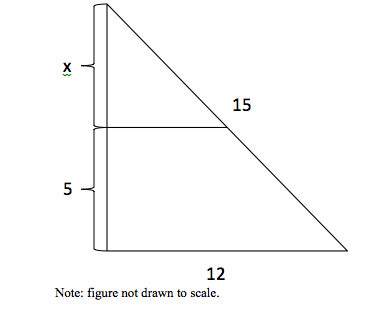5

3.5

4

4.5

5.5

4

Explanation:

Using the Pythagorean Theorem, the height of the right triangle is found to be = √(〖15〗–〖12〗2) = 9, so x=9 – 5=4

### Example Question #2 : How To Find The Length Of The Side Of A Right Triangle

A right triangle has sides of 36 and 39(hypotenuse).  Find the length of the third side

15

42

33

33√2

12 √6

15

Explanation:

use the pythagorean theorem:

a2 + b2 = c2  ; a and b are sides, c is the hypotenuse

a2 + 1296 = 1521

a2 = 225

a = 15

### Example Question #1 : How To Find The Length Of The Side Of A Right Triangle

Bob the Helicopter is at 30,000 ft. above sea level, and as viewed on a map his airport is 40,000 ft. away. If Bob travels in a straight line to his airport at 250 feet per second, how many minutes will it take him to arrive?

4 hours and 0 minutes

2 hours and 30 minutes

3 minutes and 20 seconds

1 hour and 45 minutes

3 minutes and 50 seconds

3 minutes and 20 seconds

Explanation:

Draw a right triangle with a height of 30,000 ft. and a base of 40,000 ft. The hypotenuse, or distance travelled, is then 50,000ft using the Pythagorean Theorem. Then dividing distance by speed will give us time, which is 200 seconds, or 3 minutes and 20 seconds.

### Example Question #4 : How To Find The Length Of The Side Of A Right Triangle

A right triangle has two sides, 9 and x, and a hypotenuse of 15. What is x?

13

14

10

11

12

12

Explanation:

We can use the Pythagorean Theorem to solve for x.

92 + x2 = 152

81 + x2 = 225

x2 = 144

x = 12

### Example Question #5 : How To Find The Length Of The Side Of A Right Triangle

The area of a right traingle is 42. One of the legs has a length of 12. What is the length of the other leg?Explanation: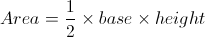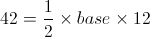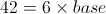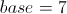### Example Question #32 : Triangles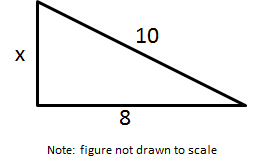Solve for x.

12

2

7

6

6

Explanation:

Use the Pythagorean Theorem. Let a = 8 and = 10 (because it is the hypotenuse)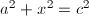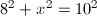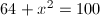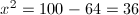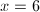### Example Question #6 : How To Find The Length Of The Side Of A Right Triangle

Solve each problem and decide which is the best of the choices given.

If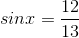, what is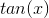?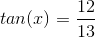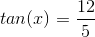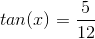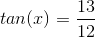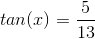Explanation:

This is a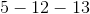triangle. We can find the value of the other leg by using the Pythagorean Theorem.

Remembering that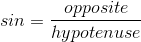.

Thus,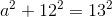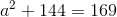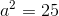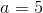.

If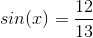, you know the adjacent side is.

Thus, making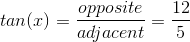because tangent is opposite/adjacent.

← Previous 1